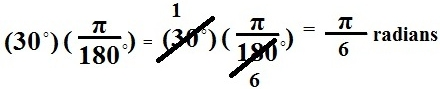﻿ How to Convert Degrees to Radians

# How to Convert from Degrees to Radians

The formula for converting from degrees to radians is below:You just put in the degrees that you want converted into radians in the formula where it says (degrees) and compute the equation and it will yield the radian value.

An example of this is below. We convert 30°into its radian value:Related Resources